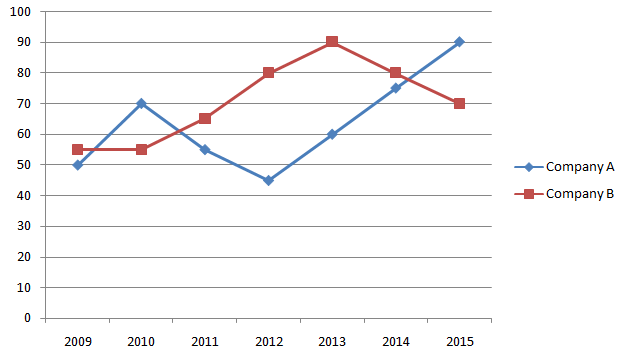#Aptitude Questions: Data Interpretation Set 63

Hello Aspirants.

Welcome to Online Quantitative Aptitude section in AffairsCloud.com. Here we are creating question sample From Data Interpretation that is important for all the competitive exams. We have included Some questions that are repeatedly asked in exams.

(Q.no: 1-10). Refer to the Graph and answer the given questions.

Percent Profit earned by Two Companies(A & B) over the years.
% Profit = [Profit Earned] / [Total Investment] * 100
Profit Earned = Total Income – Total Investment1. If the profit earned in 2011 by Company B was Rs. 5,85,000, what was the total income of the company in that year?
A. Rs. 12,50,000
B. Rs. 12,62,500
C. Rs. 16,50,000
D. Rs. 14,85,000
E. None of these
Explanation :
Income  = 585000/65 * 165 = 1485000

2. If the total amount invested by the two companies in 2014 was 30 lakh, while the amount invested by company B was 50% of the amount invested by Company A, what was the total profit earned by two companies together?
A. Rs. 23 Lakhs
B. Rs. 24 Lakhs
C. Rs. 25 Lakhs
D. Rs. 21 Lakhs
E. None of these
Explanation :
Amount invested by Company A in 2014 = 2/3 * 30,00,000 = 20 lakh
Amount invested by Company B in 2014 = 1/3 * 30,00,000 = 10 lakh
Profit earned by Company A = 75/100 * 20,00,000 = 15 lakh
Profit earned by Company B = 80/100 * 10,00,000 = 8 lakh
Total Profit = 23 lakh

3. If the amount invested by the two companies in 2010 was equal, what was the ratio of the total income of the company A to that of B in 2010?
A. 31:33
B. 33:31
C. 34:11
D. 34:31
E. None of these
Explanation :
Income of A in 2005 = 1.70x
Income of B in 2005 = 1.55x
A:B = 1.70x : 1.55x = 34 : 31

4. If the Income of Company A in 2012 and that in 2013 were equal and the amount invested in 2012 was 10 lakh, what was the amount invested in 2013?
A. Rs. 9,06,250
B. Rs. 8,06,750
C. Rs. 7,06,250
D. Rs. 5,06,750
E. None of these
Explanation :
Income of Company A in 2012 = 145/100 * 10,00,000 = 1450000
Amount invested in 2013 = 1450000 / 160 * 100 = 9,06,250

5. If the amount of profit earned by Company A in 2011 was Rs. 11.55 Lakh, what was the total investment?
A. Rs. 13 lakh
B. Rs. 15 lakh
C. Rs. 21 lakh
D. Rs. 24 lakh
E. None of these
Explanation :
55/100 * x = 11,55,000
x = 21 lakh

6. If the amount invested by Company B in 2009 is Rs. 16 lakh and the income of 2009 is equal to the investment in 2010, what is the amount of profit earned in 2010 by Company B?
A. Rs. 16.64 Lakh
B. Rs. 18.62 Lakh
C. Rs. 13.23 Lakh
D. Rs. 13.64 Lakh
E. None of these
Explanation :
Income of Company B in 2009 = 1.55 * 16,00,000 = 24.8 lakh
Investment in 2010 = 24.8 lakh
Profit earned in 2010 = 55/100 * 24,80,000 = 13.64 lakh

7. Approximately, what was the average % profit earned by Company B in all the years together?
A. 70%
B. 75%
C. 60%
D. 50%
E. None of these
Explanation :
Average percent profit = 495/7 = 70.71%

8. If the investments of the company A in 2012 and 2013 were equal, what is the difference between the profits earned in the two years if the income in 2013 was Rs.48 lakh?
A. Rs. 6.6 Lakh
B. Rs. 4.5 Lakh
C. Rs. 9.2 Lakh
D. Rs. 5.6 Lakh
E. None of these
Explanation :
Investment of Company A in 2013 = 48,00,000 / 1.60 = 30 lakh
Profit earned in 2013 = 48 – 30 = 18 lakh
Profit earned in 2014 = 45/100 * 30,00,000 = 13.50 lakh
Difference = 18 – 13.5 = 4.5 lakh

9. If each of the companies A and B invested Rs. 10 Lakh in 2015, what was the average profit earned by the two companies?
A. Rs. 8 Lakh
B. Rs. 5 Lakh
C. Rs. 7 Lakh
D. Rs. 6 Lakh
E. None of these
Explanation :
Average Profit = [(90/100 * 10,00,000) + (70/100 * 10,00,000)] / 2 = 8 lakh

10. What was the average percent profit earned by Company A in all the years together?
A. 53.57%
B. 63.57%
C. 73.57%
D. 83.57%
E. None of these